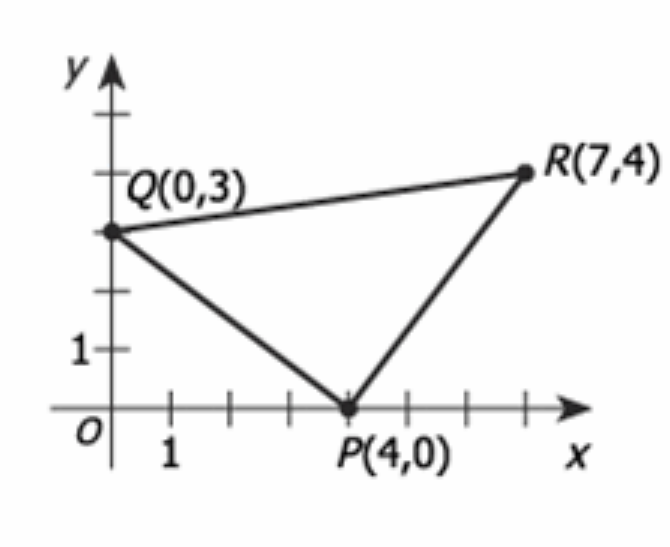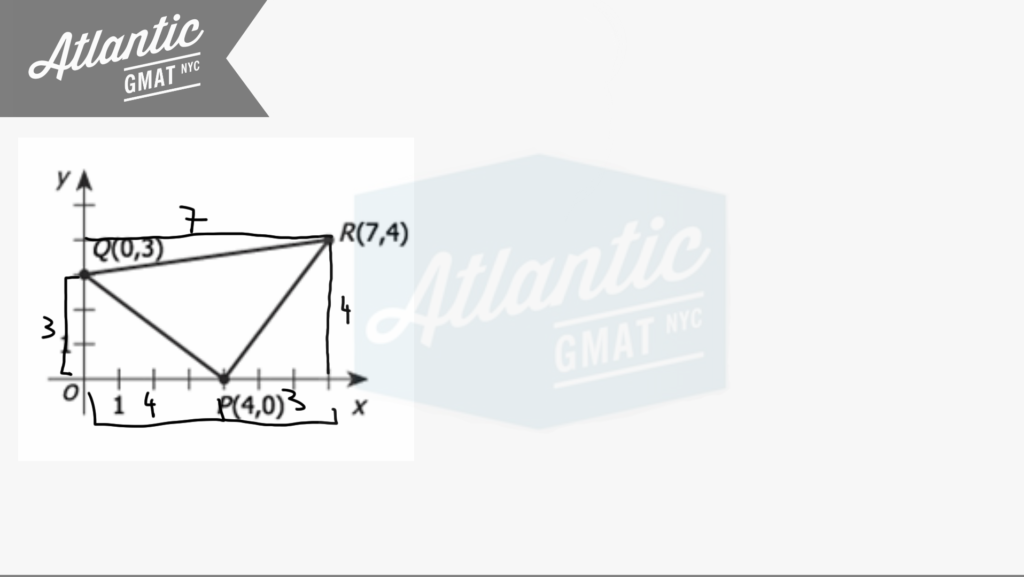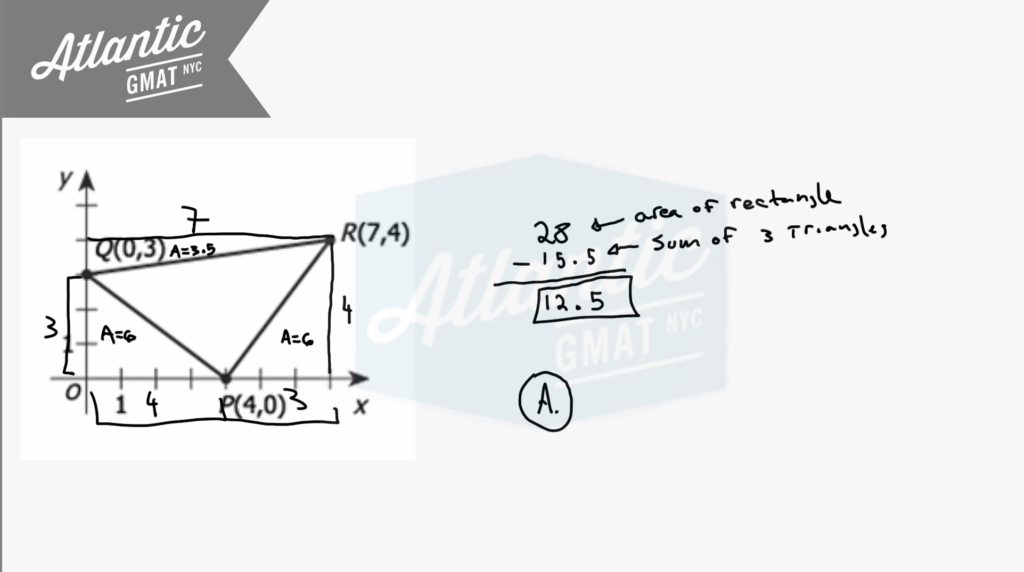In the rectangular coordinate system above, the area of triangular region PQR is

(A) 12.5

(B) 14

(C) 10√2

(D) 16

(E) 25

For GMAT geometry questions be active with diagrams. Don't be satisfied with what you're given. Try to make simple additions that provide more information (inferences). In general when trying to find an area on the coordinate plane you need to make a square, rectangle, or at the least, a right triangle. Right angles make measurement possible. Also, be open to the idea of adding/subtracting the areas of two or more shapes in order to solve for the desired area. In this case I'd draw two extra lines to make a rectangle.With that you should notice that you've created extra triangles and that those are right triangles. On GMAT geometry right triangles are your friends because you can do Pythagorean theorem and calculating are uses on the sides of the triangle (there's no need to drop an altitude). Now you can calculate the area of the rectangle and then subtract from that the areas of the three right triangles. That will leave the area of the middle triangle. Comment with any question or additions. Happy studies!To see it done live here is a video solution:

# CONTACT

Atlantic GMAT

405 East 51st St.

NY, NY 10022

(347) 669-3545

info@AtlanticGMAT.com